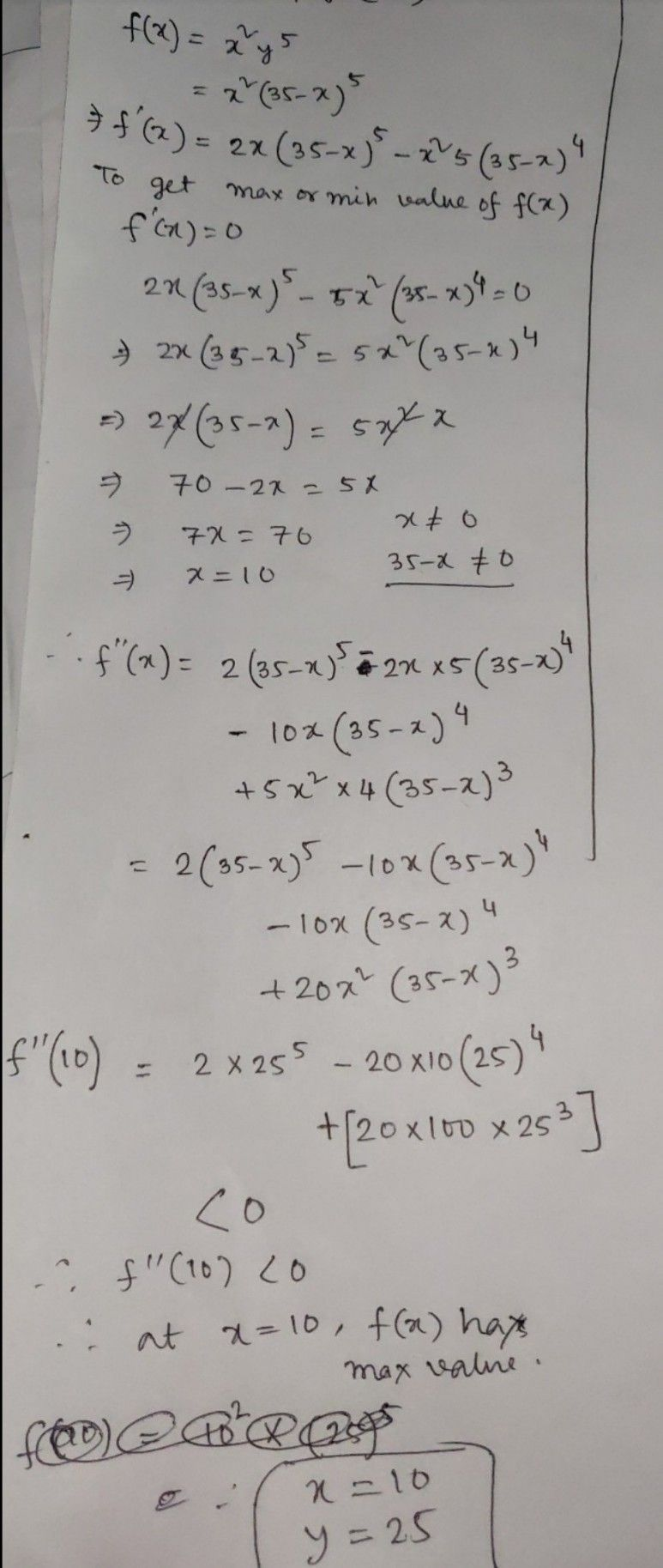Symbol
Problem$1$ $,40$ $\right)°$ $8$ $1824$ and whose product is as large as possible. $140$ Find two positive numbers $x$ and $y$ such that $x+y=60$ and $xy^{3}$ is maximum. $15.$ Find $tw0r$ positive numbers $x$ and $v$ such th
Calculus
Search count: 106
SolutionQanda teacher - Vinayadavevaluate please